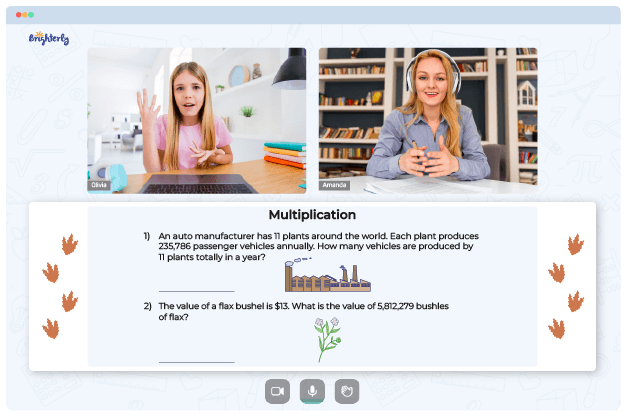# Double Digit Multiplication Worksheets

Multiplication is a fundamental operation in math for speeding up addition. As kids progress into the fourth grade, they begin to multiply numbers with double digits. But many children still think that multiplication is complicated, especially when it involves double digits. Fortunately, double-digit multiplication worksheets can help them.

## How Do Tutors at Brighterly Use Double-Digit Multiplication Worksheets?

Brighterly is an online platform with tutors who help elementary school students understand math better. When teaching multiplication, the tutors want students to understand how to apply that knowledge in solving problems with double digits and beyond. They help students commit time to practice multiplication skills using a simple 2-digit multiplication worksheet.

The math teachers at Brighterly understand that multiplication problems can look challenging at first glance, so they use fun two-digit multiplication worksheets to remove the tension. The sheets usually contain basic concepts before a student can proceed to more complex ones. They teach multiplication of double digits using simple procedures. Afterward, students then apply similar concepts to higher digits.

You can also help simplify double-digit multiplication for children by using educational worksheets that visually depict the processes. Introduce the area model, place value approach, and other tricks as practical teaching tools to help students. Using Brighterly’s strategy, you can get double-digit multiplication worksheets in PDF files for your child.

Math for Kids

Is Your Child Struggling With Math?
1:1 Online Math Tutoring## Why Double-Digit Multiplication Worksheets Are Highly Recommended

Multi-digit multiplication will be complex for students if they do not grasp the stages of the operation. Many tutors teach a seven-stage process starting with writing double-digit numbers on top of each other. Then they go through the steps multiplying single-digit numbers in each column until they get results that they add to reach a final answer.

With multiply two-digit numbers worksheets, those stages become interwoven and seamless, which makes it faster for students to get the final answer.

Helping your kid with online math lessons for 4th grade. Expert tutors inspiring kids to love math so they can excel at It.

Designers of 2-digit multiplication worksheets strive to redesign the method of teaching students as they understand that multiplying two double-digit numbers isn’t easy for kids. A double-digit multiplication worksheet showcases strategies for simplifying multiplication. Since kids can better remember things that are presented engagingly, the designers make worksheets full of colors.### Double Digit Multiplication Worksheets PDF

2 Digit Multiplication Worksheet### Double Digit Multiplication Worksheets PDF

Two Digit Multiplication Worksheets### Double Digit Multiplication Worksheets PDF

2 Digit Multiplication Worksheets### Double Digit Multiplication Worksheets PDF

Double Digit Multiplication Worksheets

The attractive designs of worksheets make students want to work with them more often. And when a child dedicates their time to constant practice of multiplication steps, they will definitely perfect the skill. By the end of the fourth grade, they won’t face any challenges multiplying two-digit numbers. And subsequently, they will have no trouble expanding their knowledge to operations with three-digit ones and more.

### More Multiplication Worksheets

Troubles with Multiplication?• Start studying with an online tutor.

Is your child finding it challenging to understand of multiplication? An online tutor could be the solution.